## Example Questions

← Previous 1 3 4 5 6 7 8

### Example Question #1 : Exponential Operations

Solve: 5x2 – 3y1 where x = 4, y = 5.

60

17

95

65

65

Explanation:

Substitute the values for x and y within the equation: 5(4)2 - 3(5)1. Proceed according to proper order of operations: 5(16) – 3(5). Therefore: 80-15= 65.

### Example Question #1 : Exponential Operations

If 64t+1 = (√2)10t + 4, what is the value of t?

1/2

–4

–1/3

1/3

4

–4

Explanation:

In order to set the exponents equal to each other and solve for t, there must be the same number raised to those exponents.

64 = (√2)n?

(√2)2 = 2 and 26 = 64, so ((√2)2)6= (√2)2*6 = (√2)12.

Thus, we now have (√2)12(t+1) = (√2)10t + 4.

12(t+1) = 10t + 4

12t + 12 = 10t + 4

2t + 12 = 4

2t = –8

t = –4

### Example Question #1 : Exponential Operations

Evaluate

(x3)2

x4

x9

x23

x6

x5

x6

Explanation:

You can simplify it into (x3)(x3) = x6

### Example Question #1 : Exponential Operations

Simplify: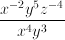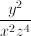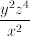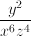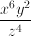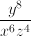Explanation:

To tackle this problem we must understand the concept of exponents in fractions and how to cancel and move them.

To move any variable or number from the numerator to the denominator or vice versa, you must negate the exponent. i.e.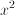in the numerator would become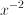in the denominator. These two expression are equivalent. You should strive to make all exponents positive initially before applying the next rule to simplify.

Cancelling variables with a similar base is an easy way to simplify. Add or subtract the exponents depending on their relationship in a fraction.

Ex.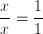or 1.

Ex.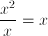. --> this can be more easily understood if you break down the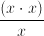which then can be moved around to form,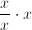. After the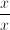cancels to form 1, we have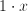or just. This can be applied for all numerical or abstract values of exponents for a given variable, such as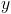or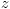.

Knowing these rules, we can tackle the problem.

To begin we will pick a variable to start with, thereby breaking down the problem into three smaller chunks. First we will start with the variable.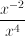. Because the numerator has a negative exponent, we will move it down to the denominator: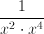. This simplifies to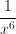as multiplying any common variables with exponents is found by addition of the exponents atop the original variable. Thevariable part of this problem is.

We move to the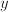section of the problem: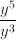. This is similar to our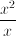above, instead with larger numerical exponents.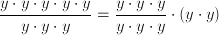. The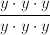section cancels, leaving us with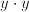or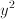.

Now to thesection. We simply have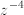on top. Applying the first rule above, we just move it to the denominator with the switching of the sign. Our result is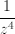.

Combining all the sections together we have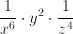.

More beautifully written it looks like.

### Example Question #2 : Exponential Operations

Simplify ((x²)-2)-3

x-12

x12

x4

x-3

x12

Explanation:

We are given an expression with a power to a power to a power. Using rules of exponents, we take the exponents and multiply each of them together.

### Example Question #3 : Exponential Operations

Find the value of x where: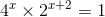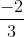Explanation: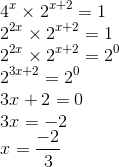### Example Question #1 : Exponential Operations

Which of the following is equivalent to: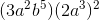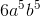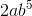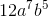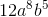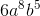Explanation:

The first step is to distribute the squared on the second term. (2a3)2 becomes 4a6 by multiplying the exponents (power raised to a power exponent rule) and squaring the 2.  Then, combining like terms (i.e. combining coefficients, a's and b's) we obtain 12a8b5.

### Example Question #2 : Exponential Operations

Simplify the following expression:

(3y2)2 + (4y)3

None of the above

9y4 + 64y3

73y12

81y4 + 64y3

73y7

9y4 + 64y3

Explanation:

This requires us to remember the rules for multiplying and adding exponent variables.

(3y2)2 can be re-written as (3)2 x (y2)2 which yields 9y4

(4y)3 can be re-written as (4)3 x (y)3 which yields 64y3

9y4 +64y3

### Example Question #3 : Exponential Operations

Simplify the following expression: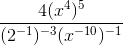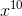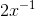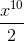Explanation:

The answer is (x10)/2. When an exponent is raised to another power, you multiply the exponents.

### Example Question #3 : Exponential Operations

Which of the following expressions is equivalent to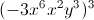?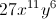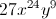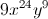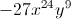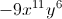Explanation:is simplified by multiplying the exponent outside of the expression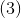, with each number and variable inside the expression: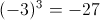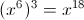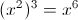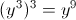This gives you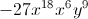When variables with exponents are multiplied, you add their respective exponents together, so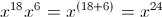Altogether, the expression is simplified to← Previous 1 3 4 5 6 7 8

### All ACT Math Resources